Engineering ToolBox - Resources, Tools and Basic Information for Engineering and Design of Technical Applications!

# Geometric Shapes - Areas

## Areas, diagonals and more - of geometric figures like rectangles, triangles, trapezoids ..

### Square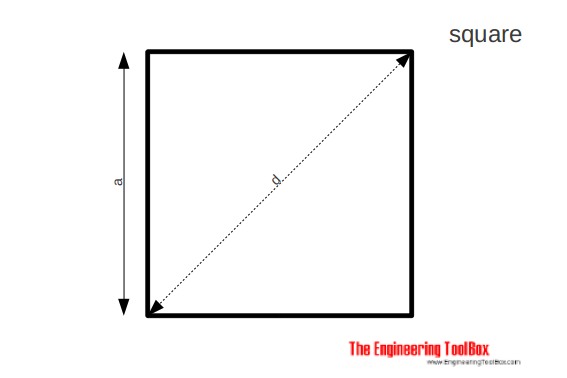The area of a square can be calculated as

A = a2                            (1a)

The side of a square can be calculated as

a = A1/2                            (1b)

The diagonal of a square can be calculated as

d = a 21/2                        (1c)

### Rectangle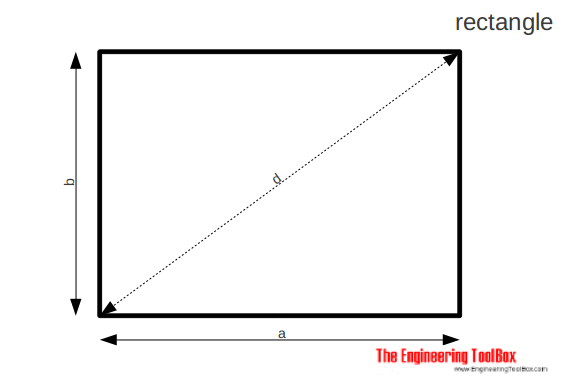The area of a rectangle can be calculated as

A = a b          (2a)

The diagonal of a rectangle can be calculated as

d = (a2 + b2)1/2     (2b)

### Parallelogram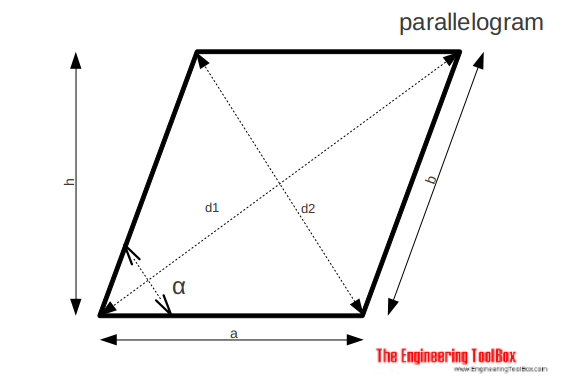The area of a parallelogram can be calculated as

A = a h

= a b sin α                        (3a)

The diameters of a parallelogram can be calculated as

d1 = ((a + h cot α)2 + h2)1/2                      (3b)

d2 = ((a - h cot α)2 + h2)1/2                     (3b)

### Equilateral Triangle

An equilateral triangle is a triangle in which all three sides are equal.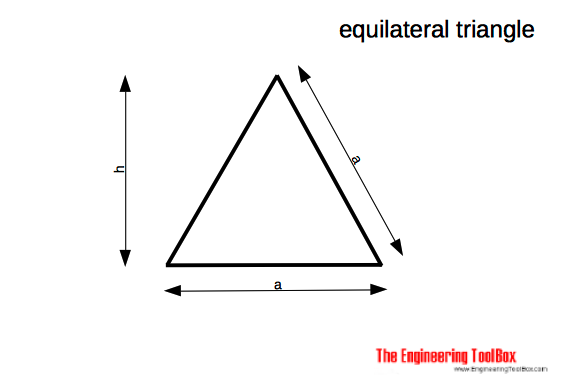The area of an equilateral triangle can be calculated as

A = a2/3 31/2                                 (4a)

The area of an equilateral triangle can be calculated as

h = a/2 31/2                              (4b)

### Triangle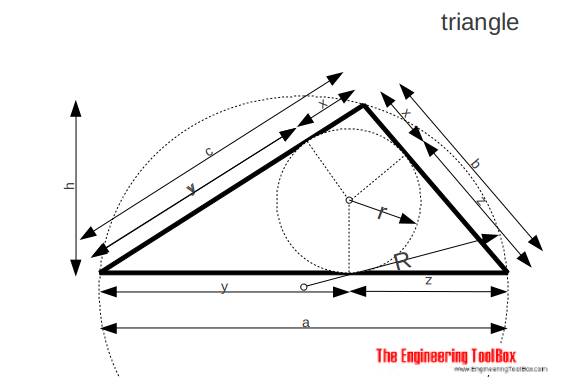The area of a triangle can be calculated as

A = a h / 2

= r s                                 (5a)

r = a h / 2s                          (5b)

R = b c / 2 h                        (5c)

s = (a + b + c) / 2                     (5d)

x = s - a                           (5e)

y = s - b                           (5f)

z = s - c                          (5g)

### Trapezoid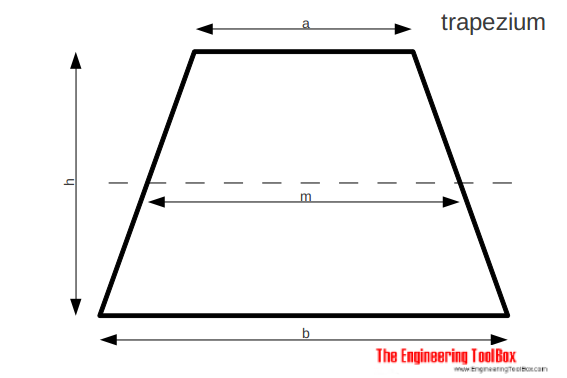The area of a trapezoid can be calculated as

A = 1/2 (a + b) h

= m h                           (6a)

m = (a + b) / 2                      (6b)

### Hexagon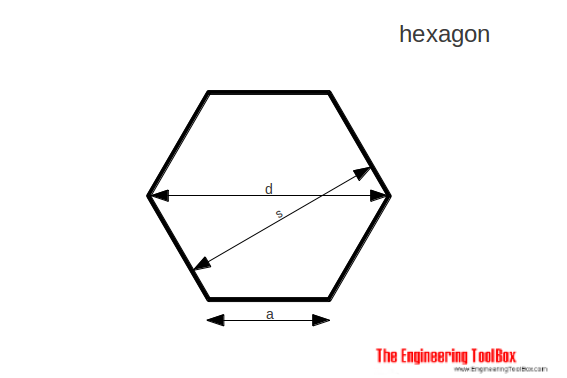The area of a hexagon can be calculated as

A = 3/2 a2 31/2                             (7a)

d = 2 a

=  2 / 31/2

= 1.1547005 s                              (7b)

s = 31/2 / 2 d

= 0.866025 d                              (7c)

### Circle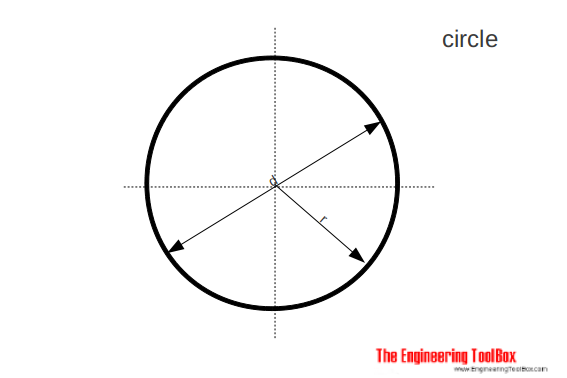The area of a circle can be calculated as

A = π/4 d2

= π r2

= 0.785.. d2                       (8a)

C = 2 π r

=  π d                           (8b)

where

C = circumference

### Sector and Segment of a Circle

#### Sector of Circle

Area of a sector of circle can be expressed as

A = 1/2 θr r2                            (9)

= 1/360 θd π r2

where

θd = angle in degrees

#### Segment of Circle

Area of a segment of circle can be expressed as

A = 1/2 (θr - sin θr) r2

= 1/2 (π θd/180 - sin θd) r2                             (10)

### Right Circular Cylinder

Lateral surface area of a right circular circle can be expressed as

A = 2 π r h                                      (11)

where

h = height of cylinder (m, ft)

r = radius of base (m, ft)

### Right Circular Cone

Lateral surface area of a right circular cone can be expressed as

A = π r l

= π r (r2 + h2)1/2                                   (12)

where

h = height of cone (m, ft)

r = radius of base (m, ft)

l = slant length (m, ft)

### Sphere

Lateral surface area of a sphere can be expressed as

A = 4 π r2                                    (13)

## Related Topics

• Mathematics - Mathematical rules and laws - numbers, areas, volumes, exponents, trigonometric functions and more.

## Engineering ToolBox - SketchUp Extension - Online 3D modeling!

Add standard and customized parametric components - like flange beams, lumbers, piping, stairs and more - to your Sketchup model with the Engineering ToolBox - SketchUp Extension - enabled for use with the amazing, fun and free SketchUp Make and SketchUp Pro .Add the Engineering ToolBox extension to your SketchUp from the SketchUp Pro Sketchup Extension Warehouse!

Translate

## Privacy

We don't collect information from our users. Only emails and answers are saved in our archive. Cookies are only used in the browser to improve user experience.

Some of our calculators and applications let you save application data to your local computer. These applications will - due to browser restrictions - send data between your browser and our server. We don't save this data.

## Citation

• Engineering ToolBox, (2008). Geometric Shapes - Areas. [online] Available at: https://www.engineeringtoolbox.com/area-geometric-figures-d_1250.html [Accessed Day Mo. Year].

Modify access date.

. .

#### Scientific Online Calculator3 30